# Cone side

Calculate the volume and area of the cone whose height is 10 cm and the axial section of the cone has an angle of 30 degrees between height and the cone side.

Result

V =  349.066 cm3
S =  314.159 cm2

#### Solution:

$h=10 \ \text{cm} \ \\ A=30 ^\circ \rightarrow\ \text{rad}=30 ^\circ \cdot \ \dfrac{ \pi }{ 180 } \ =30 ^\circ \cdot \ \dfrac{ 3.1415926 }{ 180 } \ =0.5236=π/6 \ \\ \ \\ \tan A=r:h \ \\ \ \\ r=h \cdot \ \tan(A)=10 \cdot \ \tan(0.5236) \doteq 5.7735 \ \text{cm} \ \\ \ \\ S_{1}=\pi \cdot \ r^2=3.1416 \cdot \ 5.7735^2 \doteq 104.7198 \ \text{cm}^2 \ \\ \ \\ V=\dfrac{ 1 }{ 3 } \cdot \ S_{1} \cdot \ h=\dfrac{ 1 }{ 3 } \cdot \ 104.7198 \cdot \ 10 \doteq 349.0659 \doteq 349.066 \ \text{cm}^3$
$s=\sqrt{ h^2+r^2 }=\sqrt{ 10^2+5.7735^2 } \doteq 11.547 \ \text{cm} \ \\ S_{2}=\pi \cdot \ r \cdot \ s=3.1416 \cdot \ 5.7735 \cdot \ 11.547 \doteq 209.4395 \ \text{cm}^2 \ \\ S=S_{1}+S_{2}=104.7198+209.4395 \doteq 314.1593 \doteq 314.159 \ \text{cm}^2$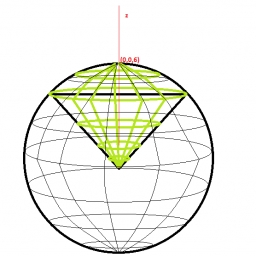Our examples were largely sent or created by pupils and students themselves. Therefore, we would be pleased if you could send us any errors you found, spelling mistakes, or rephasing the example. Thank you!

Leave us a comment of this math problem and its solution (i.e. if it is still somewhat unclear...):Be the first to comment!Tips to related online calculators
Check out our ratio calculator.
Tip: Our volume units converter will help you with the conversion of volume units.
Pythagorean theorem is the base for the right triangle calculator.

## Next similar math problems:

1. Squares ratioThe first square has a side length of a = 6 cm. The second square has a circumference of 6 dm. Calculate the proportions of the perimeters and the proportions of the contents of these squares? (Write the ratio in the basic form). (Perimeter = 4 * a, conte
2. Five-gon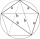Calculate the side a, the circumference and the area of the regular 5-angle if Rop = 6cm.
3. Triangular prismThe base of the perpendicular triangular prism is a rectangular triangle with a hypotenuse of 10 cm and one leg of 8 cm. The prism height is 75% of the perimeter of the base. Calculate the volume and surface of the prism.
4. Right angleIf a, b and c are two sides of a triangle ABC, a right angle in A, find the value on each missing side. If b=10, c=6
5. Corona virus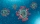Calculate an estimate of the percentage of GDP (Gross Domestic Product) declined due to the quarantine related to the corona COVID-19 virus that lasts for 2 months, during which time the economic activity is down by 80%.
6. Compute 4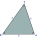Compute the exact value of the area of the triangle with sides 14 mi, 12 mi, and 12 mi long.
7. KostkaKostka je vepsána do koule o poloměru r = 6 cm. Kolik procent tvoří objem kostky z objemu koule?
8. Cuboid and ratioFind the dimensions of a cuboid having a volume of 810 cm3 if the lengths of its edges coming from the same vertex are in ratio 2: 3: 5
9. Wall thicknessThe hollow metal ball has an outside diameter of 40 cm. Determine the wall thickness if the weight is 25 kg and the metal density is 8.45 g/cm3.
10. The surface areaHow much percent will the surface area of a 4x5x8 cm block increase if the length of the shortest edge is increased by 2 cm?
11. What percentageWhat percentage of the Earth’s surface is seen by an astronaut from a height of h = 350 km. Take the Earth as a sphere with the radius R = 6370 km
12. Corona again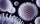If the population on long island is 7.75 million and the coronavirus has affected 22,178. What percent of the population is affected. (looking for 1 in? If possible)
13. Sum of the seventeen numbersThe sum of the 17 different natural numbers is 154. Determine the sum of the two largest ones.
14. Fall sum or sameFind the probability that if you roll two dice, it will fall the sum of 10, or the same number will fall on both dice.
15. AnnulusTwo concentric circles with radii 1 and 9 surround the annular circle. This ring is inscribed with n circles that do not overlap. Determine the highest possible value of n.
16. What percent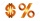What percent of 51 3/5 is 47 2/5?
17. Self-oscillation period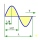The water in the vessel carried by the boy has a self-oscillation period of 0.8 s. What is the size of the boy's movement speed when the length of the boy's step is 60 cm? Give the result in m/s.